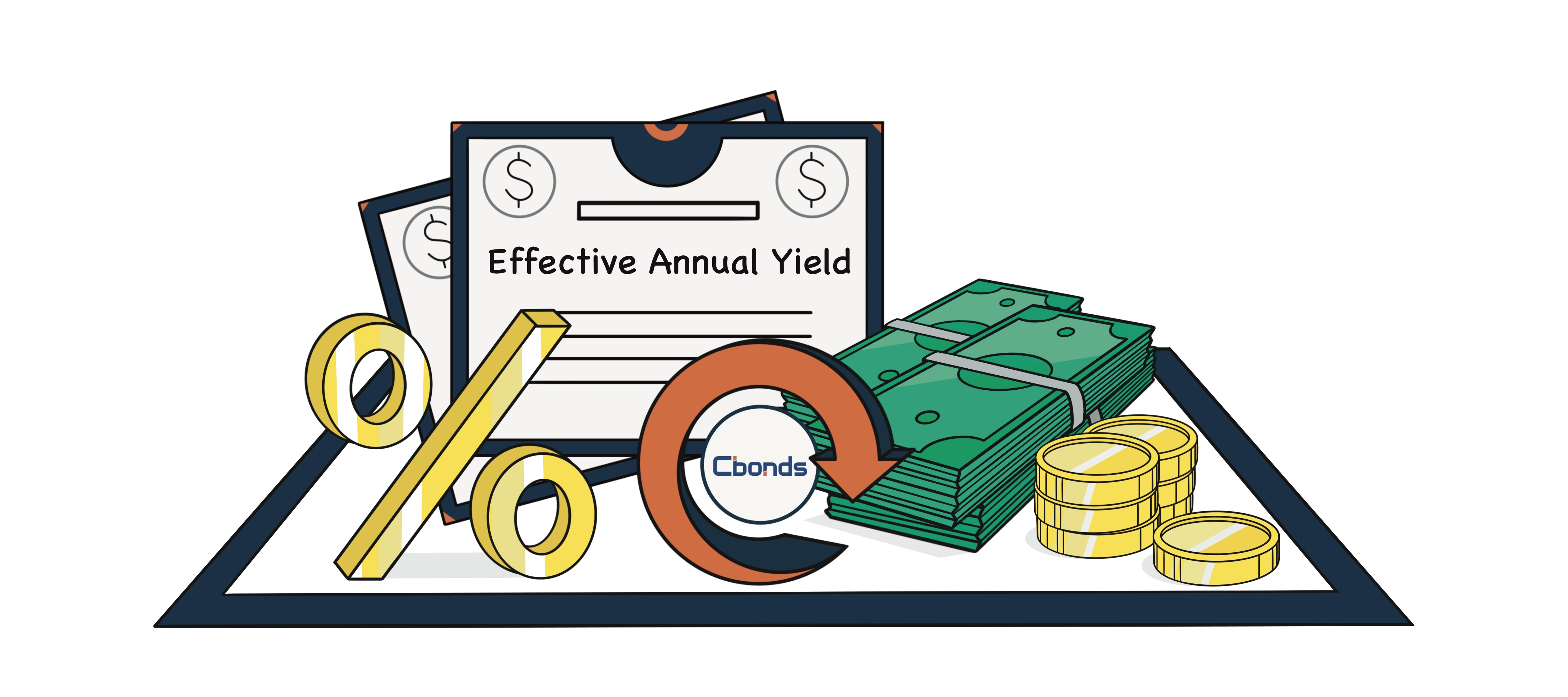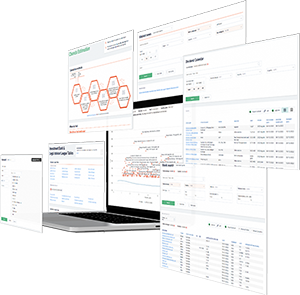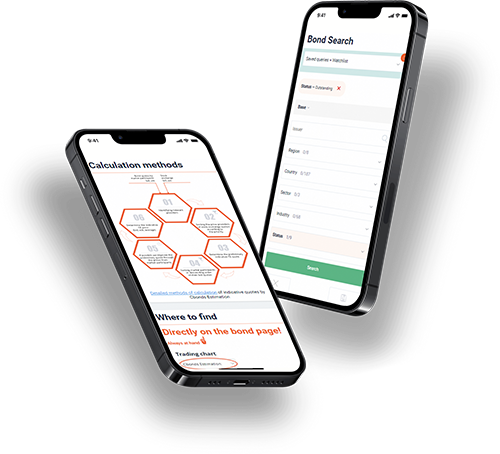Hint mode is switched on
Glossary

# Effective Annual Yield

Category — Analytical Metrics

## What Is the Effective Yield?

The effective yield represents an investor’s total return on a bond when the interest payments, also known as coupons, are reinvested at the same interest rate. Unlike the nominal yield, which merely indicates the stated interest rate of the bond’s coupon, effective yield takes into consideration the compounding effect on investment returns. By acknowledging the power of compounding, effective yield provides a more comprehensive measure of the actual yield received by the investor.

It is important to note that there is no distinction between "effective yield" and "effective annual yield" as two separate concepts in financial terminology. Both terms are often used interchangeably to refer to the same measure of the annualized rate of return, which considers compounding effects.

Therefore, in practice, "effective yield" and "effective annual yield" generally refer to the same concept: the annualized return on an investment that incorporates compounding effects.## Understanding Effective Annual Yield

The effective yield serves as a metric for assessing the coupon rate, denoting the stated interest rate of a bond expressed as a percentage of its face value. Bond issuers typically make coupon payments to bond investors twice a year, resulting in two coupon payments annually. To calculate the effective yield, one divides the coupon payments by the bond’s current market value.

Effective yield gives bondholders a means to gauge the returns on their bond investments. Another measure to consider is the current yield, which represents the annual return on a bond based on its annual coupon payments and current price rather than its face value.

## Effective Annual Yield Formula

The effective annual yield (EAY) is calculated using the following formula:

EAY = (1 + (r/n))^n - 1

Where:

• "r" represents the nominal rate or an annualized rate of return.

• "n" represents the number of compounding periods within one year.

This formula assumes that the interest or investment gains are compounded at regular intervals throughout the year. By plugging in the values for the nominal interest rate and the number of compounding periods, the formula calculates the effective annual yield, which represents the true annualized return on the investment, accounting for compounding effects.

## Calculating Effective Annual Yield

To calculate the effective yield, follow the steps outlined below:

1. Determine the number of payments (n) received during the year. For securities that pay semi-annually (every 6 months), set n as 2. Likewise, quarterly payments would correspond to n = 4, and monthly payments would have n = 12.

2. Identify the stated rate of interest (ROI), denoted as ’i’, already provided for the financial security.

3. Divide the rate of interest (i) by the number of payment intervals (n) determined in Step 1, considering the interest rate in decimal form.

4. Calculate the sum of 1 + (i/n).

5. Raise the value obtained in Step 4 to the power of ’n’.

6. Deduct 1 from the result obtained in Step 5 to derive the annualized yield.

By following these steps, you can determine the effective yield, providing a comprehensive measure of the annualized return on financial security.

## Using Effective Annual Yield to Compare Bonds

To compare the effective yield and yield-to-maturity of a bond, it is necessary to convert the effective yield into an effective annual yield. Bonds with an effective yield higher than the yield-to-maturity are typically sold at a premium. Conversely, if the effective yield is lower than the yield-to-maturity, the bond is likely to trade at a discount. This comparison between the two yields provides valuable insights into bond pricing and market positioning.

## Effective Yield vs. Yield-to-Maturity (YTM)

The yield-to-maturity (YTM) represents the rate of return obtained on a bond held until its maturity date. To compare the effective yield and YTM, it is essential to convert the YTM into an effective annual yield. If the YTM surpasses the bond’s effective yield, the bond is considered to be trading at a discount to its par value. Conversely, if the YTM falls below the effective yield, the bond is selling at a premium.

YTM is classified as a bond equivalent yield (BEY), giving investors an approximate annual yield. However, to obtain a more accurate annual yield, investors can calculate the effective annual yield (EAY) by incorporating the time value of money into the calculation. This enables investors to account for the compounding effect and obtain a more precise measure of the bond’s actual annual yield.

## Effective Yield and Bond Equivalent Yield

The bond equivalent yield can be readily converted into the annual effective yield by multiplying it by the number of days in a year. For instance, if the bond equivalent yield is 8%, then the annual effective yield would be 8% multiplied by 365, resulting in 29%.

The conversion from bond equivalent yield to annual effective yield is a straightforward process that allows investors to assess the true annual return on their investments. Investors can accurately calculate the effective yield by considering the compounding periods and using the effective annual yield formula. This is particularly useful when dealing with different types of securities, such as zero-coupon bonds, where interest is not paid annually but rather upon maturity.

Get access
×

## — We have everything you need:full data on over 700 000 bonds, stocks & ETFs; powerful bond screener; over 350 pricing sources among stock exchanges & OTC market; ratings & financial reports; user-friendly interface; available anywhere via Website, Excel Add-in and Mobile app.

×

## WhyYou will have detailed descriptive & pricing data for 650K bonds, 76K stocks, 8K ETFsGet full access to the platform from any device & via Cbonds app